# 20 行代码：Serverless 架构下用 Python 轻松搞定图像分类和预测## 前言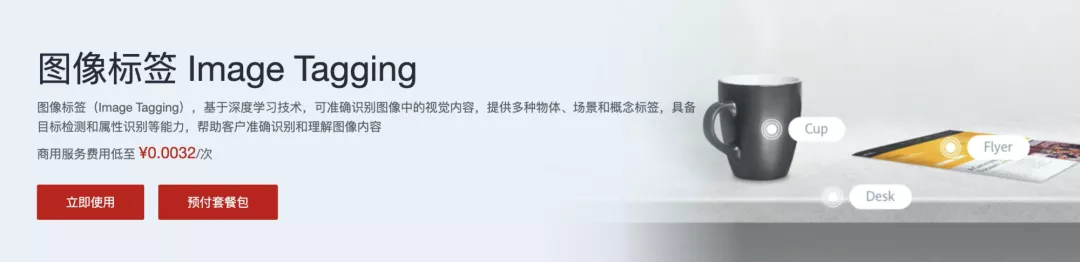ImageAI 是一个 python 库，旨在使开发人员能够使用简单的几行代码构建具有包含深度学习和计算机视觉功能的应用程序和系统。
ImageAI 本着简洁的原则，支持最先进的机器学习算法，用于图像预测、自定义图像预测、物体检测、视频检测、视频对象跟踪和图像预测训练。ImageAI 目前支持使用在 ImageNet-1000 数据集上训练的 4 种不同机器学习算法进行图像预测和训练。ImageAI 还支持使用在 COCO 数据集上训练的 RetinaNet 进行对象检测、视频检测和对象跟踪。最终，ImageAI 将为计算机视觉提供更广泛和更专业化的支持，包括但不限于特殊环境和特殊领域的图像识别。

``````# -*- coding: utf-8 -*-
from imageai.Prediction import ImagePrediction

# 模型加载
prediction = ImagePrediction()
prediction.setModelTypeAsResNet()
prediction.setModelPath("resnet50_weights_tf_dim_ordering_tf_kernels.h5")

predictions, probabilities = prediction.predictImage("./picture.jpg", result_count=5 )
for eachPrediction, eachProbability in zip(predictions, probabilities):
print(str(eachPrediction) + " : " + str(eachProbability))``````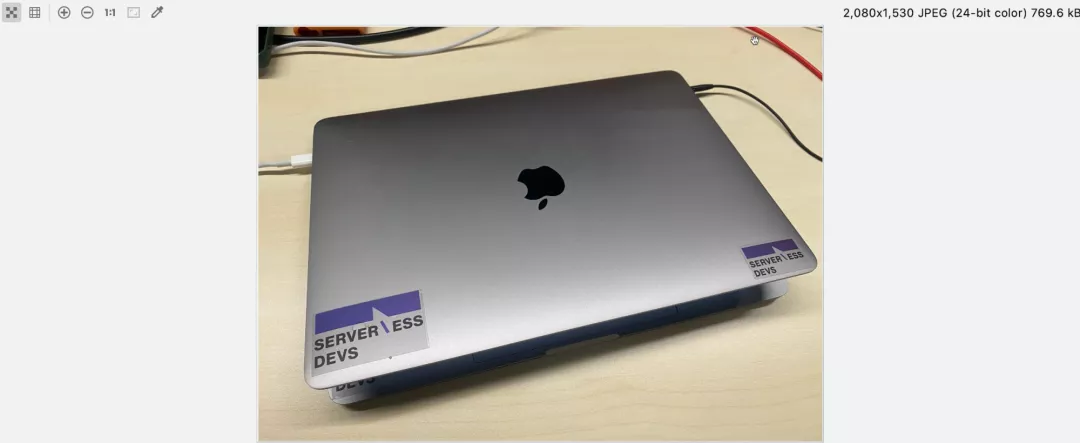``````laptop : 71.43893241882324
notebook : 16.265612840652466
modem : 4.899394512176514
hard_disc : 4.007557779550552
mouse : 1.2981942854821682``````

• SqueezeNet（文件大小：4.82 MB，预测时间最短，精准度适中）
• ResNet50 by Microsoft Research （文件大小：98 MB，预测时间较快，精准度高）
• InceptionV3 by Google Brain team （文件大小：91.6 MB，预测时间慢，精度更高）
• DenseNet121 by Facebook AI Research （文件大小：31.6 MB，预测时间较慢，精度最高）

https://github.com/OlafenwaMoses/ImageAI/releases/tag/1.0

## 项目 Serverless 化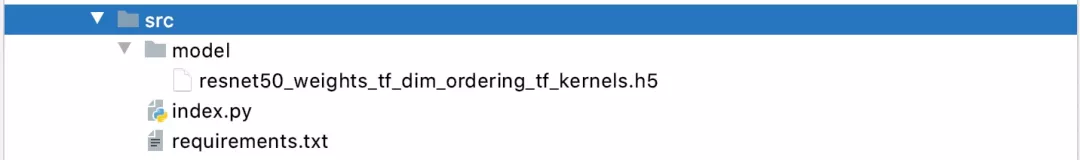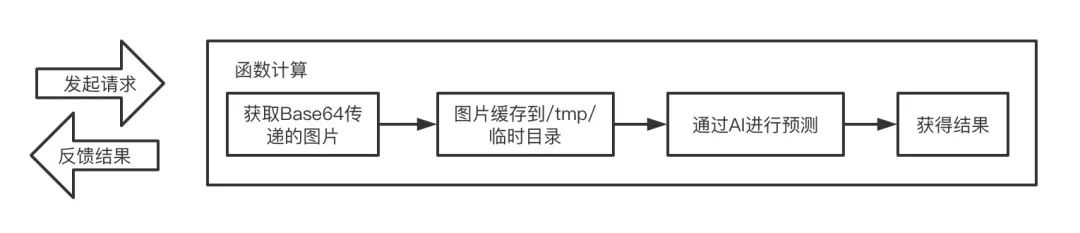``````# -*- coding: utf-8 -*-

from imageai.Prediction import ImagePrediction
import json
import uuid
import base64
import random

# Response
class Response:
def __init__(self, start_response, response, errorCode=None):
self.start = start_response
responseBody = {
'Error': {"Code": errorCode, "Message": response},
} if errorCode else {
'Response': response
}
# 默认增加uuid，便于后期定位
responseBody['ResponseId'] = str(uuid.uuid1())
print("Response: ", json.dumps(responseBody))
self.response = json.dumps(responseBody)

def __iter__(self):
status = '200'
yield self.response.encode("utf-8")

# 随机字符串
randomStr = lambda num=5: "".join(random.sample('abcdefghijklmnopqrstuvwxyz', num))

# 模型加载
print("Init model")
prediction = ImagePrediction()
prediction.setModelTypeAsResNet()
prediction.setModelPath("/mnt/auto/model/resnet50_weights_tf_dim_ordering_tf_kernels.h5")

def handler(environ, start_response):
try:
request_body_size = int(environ.get('CONTENT_LENGTH', 0))
except (ValueError):
request_body_size = 0

# 图片获取
print("Get pucture")
imageName = randomStr(10)
imageData = base64.b64decode(requestBody["image"])
imagePath = "/tmp/" + imageName
with open(imagePath, 'wb') as f:
f.write(imageData)

# 内容预测
print("Predicting ... ")
result = {}
predictions, probabilities = prediction.predictImage(imagePath, result_count=5)
print(zip(predictions, probabilities))
for eachPrediction, eachProbability in zip(predictions, probabilities):
result[str(eachPrediction)] = str(eachProbability)

return Response(start_response, result)``````

``````tensorflow==1.13.1
numpy==1.19.4
scipy==1.5.4
opencv-python==4.4.0.46
pillow==8.0.1
matplotlib==3.3.3
h5py==3.1.0
keras==2.4.3
imageai==2.1.5``````

``````ServerlessBookImageAIDemo:
Component: fc
Provider: alibaba
Access: release
Properties:
Region: cn-beijing
Service:
Name: ServerlessBook
Description: Serverless图书案例
Log: Auto
Nas: Auto
Function:
Name: serverless_imageAI
Description: 图片目标检测
CodeUri:
Src: ./src
Excludes:
- src/.fun
- src/model
Handler: index.handler
Environment:
- Key: PYTHONUSERBASE
Value: /mnt/auto/.fun/python
MemorySize: 3072
Runtime: python3
Timeout: 60
Triggers:
- Name: ImageAI
Type: HTTP
Parameters:
AuthType: ANONYMOUS
Methods:
- GET
- POST
- PUT
Domains:
- Domain: Auto``````

## 项目部署与测试

``s deploy``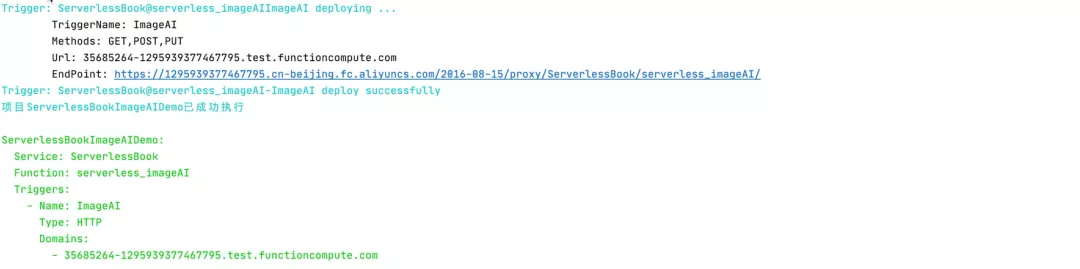``s install docker``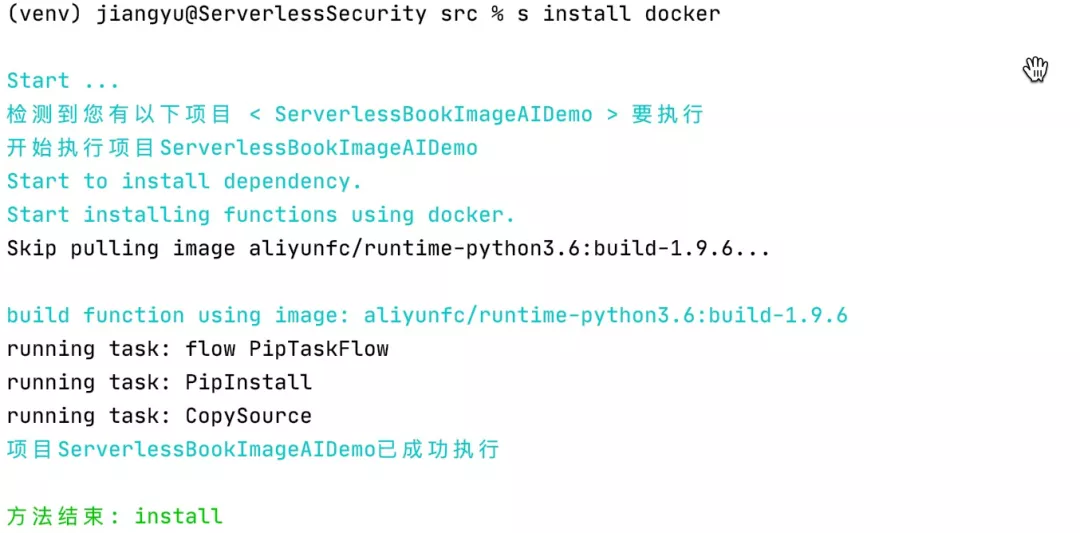``s nas sync ./src/.fun``

``s nas sync ./src/model``

``s nas ls --all``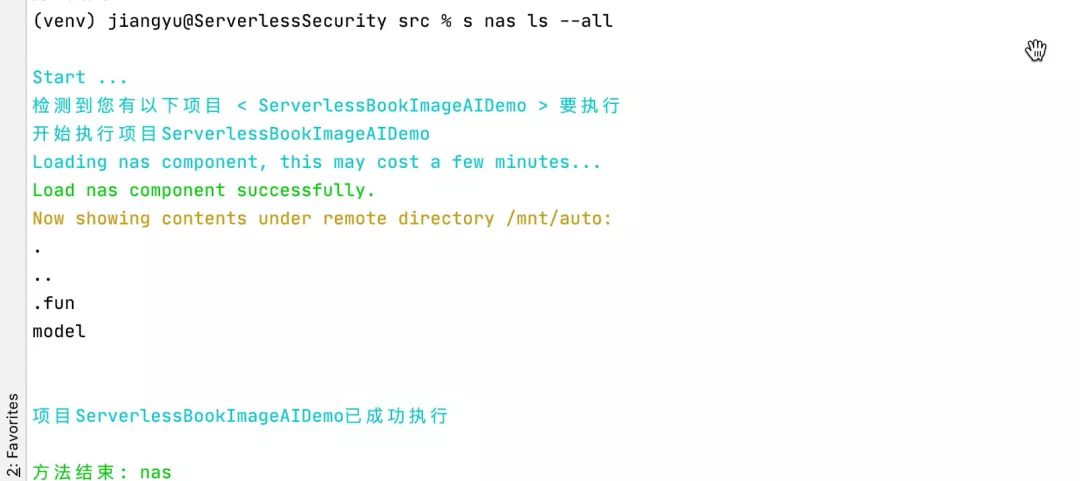``````import json
import urllib.request
import base64
import time

with open("picture.jpg", 'rb') as f:

url = 'http://35685264-1295939377467795.test.functioncompute.com/'

timeStart = time.time()
print(urllib.request.urlopen(urllib.request.Request(
url=url,
data=json.dumps({'image': data}).encode("utf-8")
print("Time: ", time.time() - timeStart)``````

``````{"Response": {"laptop": "71.43893837928772", "notebook": "16.265614330768585", "modem": "4.899385944008827", "hard_disc": "4.007565602660179", "mouse": "1.2981869280338287"}, "ResponseId": "1d74ae7e-298a-11eb-8374-024215000701"}
Time:  29.16020894050598``````

``````{"Response": {"laptop": "71.43893837928772", "notebook": "16.265614330768585", "modem": "4.899385944008827", "hard_disc": "4.007565602660179", "mouse": "1.2981869280338287"}, "ResponseId": "4b8be48a-298a-11eb-ba97-024215000501"}
Time:  1.1511380672454834``````

## 项目优化

``````# -*- coding: utf-8 -*-

import time

timeStart = time.time()

# 模型加载
from imageai.Prediction import ImagePrediction

prediction = ImagePrediction()
prediction.setModelTypeAsResNet()
prediction.setModelPath("resnet50_weights_tf_dim_ordering_tf_kernels.h5")
print("Load Time: ", time.time() - timeStart)
timeStart = time.time()

predictions, probabilities = prediction.predictImage("./picture.jpg", result_count=5)
for eachPrediction, eachProbability in zip(predictions, probabilities):
print(str(eachPrediction) + " : " + str(eachProbability))
print("Predict Time: ", time.time() - timeStart)``````

``````Load Time:  5.549695014953613
laptop : 71.43893241882324
notebook : 16.265612840652466
modem : 4.899394512176514
hard_disc : 4.007557779550552
mouse : 1.2981942854821682
Predict Time:  0.8137111663818359``````

## 总结

• Serverless 架构可以运行人工智能相关项目；
• Serverless 可以很好地兼容 Tensorflow 等机器学习/深度学习的工具；
• 虽然说函数计算本身有空间限制，但是实际上增加了硬盘挂载能力之后，函数计算本身的能力将会得到大幅度的拓展。